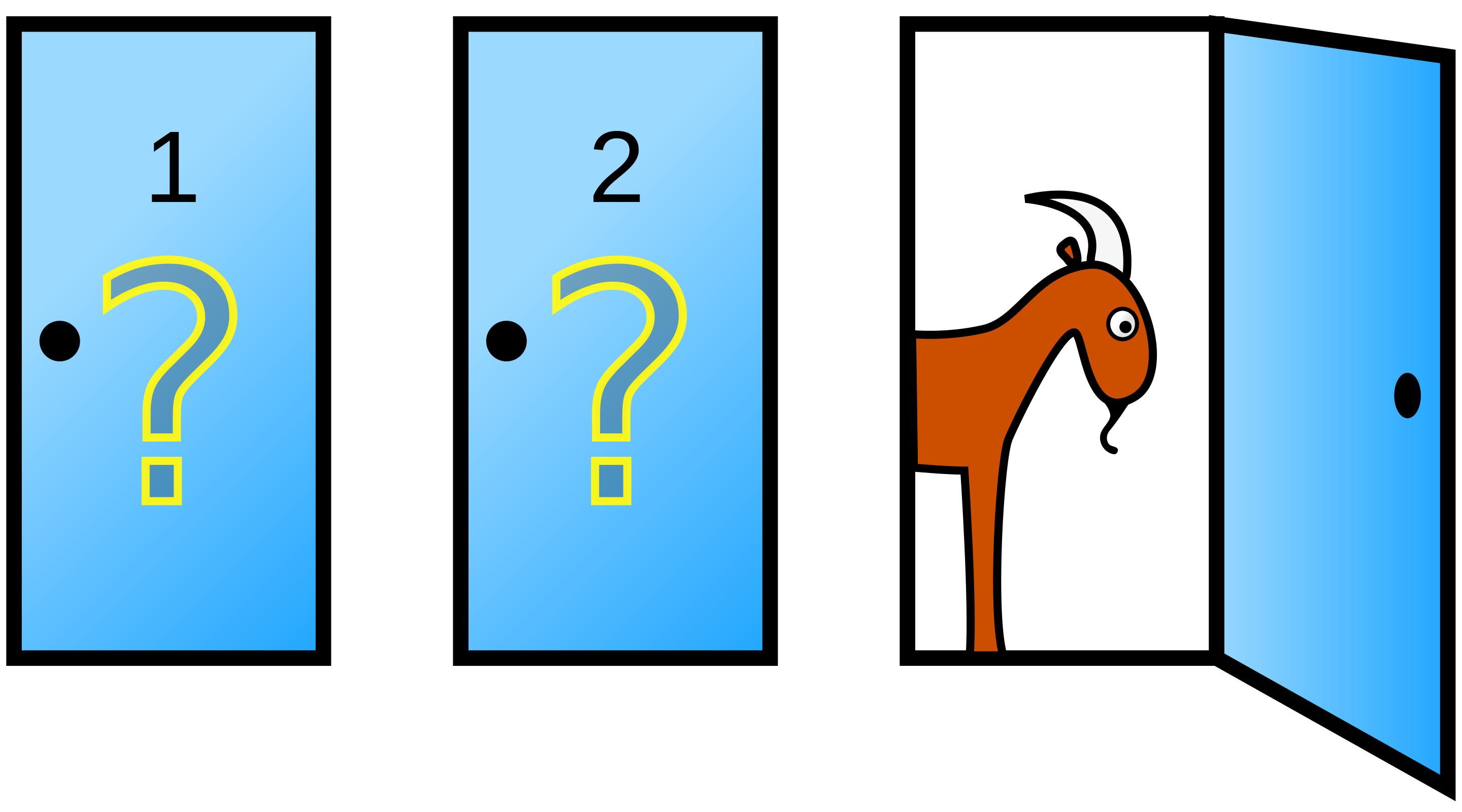# Monty Hall problem

### To change or not to change doors?Most people familiar with the Monty Hall problem find it counter-intuitive that we should always accept the offer to change doors in the game, as that gives us a ~66% probability of success in the long run (vs. ~33% if we don’t change doors). You can read the Wiki article linked below to understand the logic behind it, but you can also simulate 300 attempts (x-axis) at the game to see how never changing vs. always changing doors affects the probability of success (y-axis).

The function in the code below, `mHall()`, is a simple simulation of the game (no arguments needed).

Code
``````mHall = function(){

options = c("money", "goat", "goat")
doors = sample(x = options, size = 3)
names(doors) = c("A", "B", "C")

firstChoice = readline(prompt = "Which door do you choose: A, B, or C? ")

if(doors[firstChoice] == "money"){
newOffer = sample(x = badDoors, size = 1)
secondChoice = readline(prompt = paste("I can tell you that door ", names(newOffer),
" has a ",
doors[names(newOffer)],
". Would you like to change your answer (y or n)? ",
sep = ""))
if(secondChoice == "y"){
newChoice = doors[!names(doors) %in% c(firstChoice, names(newOffer))]
return(paste("Here's what's behind door ", names(newChoice), ": ",
newChoice[], sep = ""))
} else {
return(paste("Here's what's behind door ", firstChoice, ": ",
doors[[firstChoice]], sep = ""))
}
} else {
notChosen = doors[!names(doors) %in% firstChoice]
goodDoor = doors[names(which(notChosen == "money"))]
secondChoice = readline(prompt = paste("I can tell you that door ", names(badDoor),
". Would you like to change your answer (y or n)? ",
sep = ""))
if(secondChoice == "y"){
newChoice = goodDoor
return(paste("Here's what's behind door ", names(newChoice), ": ",
newChoice[], sep = ""))
} else {
return(paste("Here's what's behind door ", firstChoice, ": ",
doors[[firstChoice]], sep = ""))
}
}

return(doors[firstChoice])
}``````